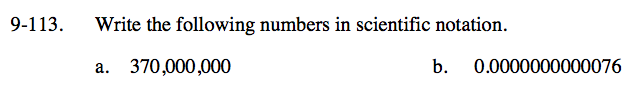### Home > CC3 > Chapter 9 > Lesson 9.2.4 > Problem9-113

9-113.
1. Write the following numbers in scientific notation. Homework Help ✎

1. 370,000,000

2. 0.0000000000076The decimal point needs to be one spot after the first nonzero number.

How many places to the left did you move the decimal? That number will be the power of ten you multiply your new number by. If you moved the decimal to the right, the exponent will be negative.

3.7 x 108

See the help for part (a).